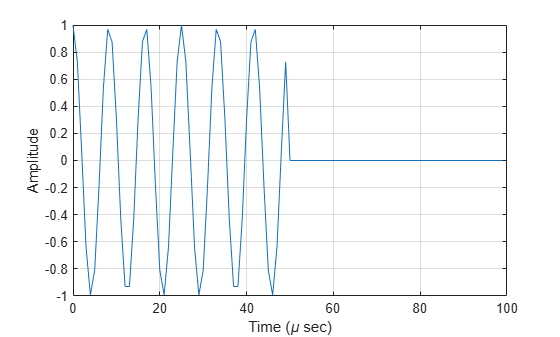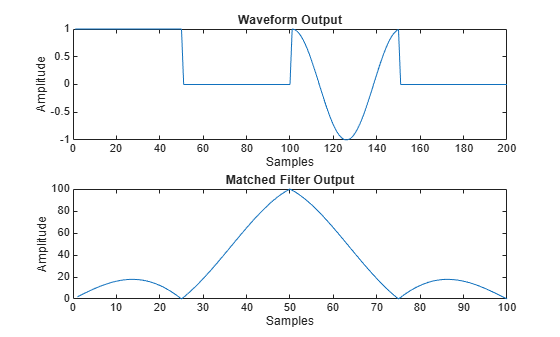# step

System object: phased.SteppedFMWaveform
Package: phased

Samples of stepped FM pulse waveform

## Syntax

Y = step(sSFM) Y = step(sSFM,prfidx) Y = step(sRFM,freqoffset) [Y,PRF] = step(___) [Y,COEFF] = step(___) 

## Description

Note

Starting in R2016b, instead of using the step method to perform the operation defined by the System object™, you can call the object with arguments, as if it were a function. For example, y = step(obj,x) and y = obj(x) perform equivalent operations. When the only argument to the step method is the System object itself, replace y = step(obj) by y = obj().

Y = step(sSFM) returns samples of the stepped FM pulses in a column vector, Y. The output, Y, results from increasing the frequency of the preceding output by an amount specified by the FrequencyStep property. If the total frequency increase is larger than the value specified by the SweepBandwidth property, the samples of a rectangular pulse are returned.

Y = step(sSFM,prfidx), uses the prfidx index to select the PRF from the predefined vector of values specified by in the PRF property. This syntax applies when you set the PRFSelectionInputPort property to true.

Y = step(sRFM,freqoffset), uses the freqoffset to generate the waveform with an offset as specified at step time. Use this syntax for cases where the transmit pulse frequency needs to be dynamically updated. This syntax applies when you set the FrequencyOffsetSource property to 'Input port'.

[Y,PRF] = step(___) also returns the current pulse repetition frequency, PRF. To enable this syntax, set the PRFOutputPort property to true and set the OutputFormat property to 'Pulses'.

[Y,COEFF] = step(___) returns the matched filter coefficients, COEFF, for the current pulse. To enable this syntax, set CoefficientsOutputPort to true. COEFF is returned as either an NZ-by-1 vector or an NZ-by-M matrix.

• An NZ-by-1 vector is returned when the object has OutputFormat set to 'Pulses' and NumPulses is equal to 1. NZ is the pulse width.

• An NZ-by-M matrix is returned when either OutputFormat set to 'Pulses' and NumPulses is greater than 1, or OutputFormat is set to 'Samples'.

• When the object generates a constant pulse width waveform (DurationSpecification set to 'Pulse width' or 'Duty cycle' and PRF has one unique value), NZ is the pulse width and M is the number of sub-pulses, NumSteps.

• When the object generates a varying pulse width waveform (DurationSpecification is set to 'Duty cycle' and PRF has more than one unique value), NZ is the maximum of the pulse width and M is the product of NumSteps and the number of unique PRFs.

You can combine optional input and output arguments when their enabling properties are set. Optional inputs and outputs must be listed in the same order as the order of the enabling properties. For example, [Y,PRF,COEFF] = step(sRFM,prfidx,freqoffset).

Note

The object performs an initialization the first time the object is executed. This initialization locks nontunable properties and input specifications, such as dimensions, complexity, and data type of the input data. If you change a nontunable property or an input specification, the System object issues an error. To change nontunable properties or inputs, you must first call the release method to unlock the object.

## Examples

expand all

Create a stepped frequency pulse waveform object with a frequency step of 40 kHz and four frequency steps.

waveform = phased.SteppedFMWaveform(... 'NumSteps',4,'FrequencyStep',40e3,... 'OutputFormat','Pulses','NumPulses',1); fs = waveform.SampleRate;

Use the waveform method to obtain the pulses.

First, generate pulse 1.

pulse1 = waveform();

Then, generate pulse 2, incremented by the frequency step 40 kHz.

pulse2 = waveform();

Next, generate pulse 3, incremented by the frequency step 40 kHz.

pulse3 = waveform();

Finally, generate pulse 4, incremented by the frequency step 40 kHz.

pulse4 = waveform(); nsamps = size(pulse4,1); t = [0:(nsamps-1)]/fs*1e6; plot(t,real(pulse4)) xlabel('Time (\mu sec)') ylabel('Amplitude') gridGenerate output samples and matched filter coefficients of a stepped FM pulse waveform.

waveform = phased.SteppedFMWaveform('NumSteps',2,'NumPulses',1,... 'CoefficientsOutputPort',true,'PRF',[1e4 1e4 2e4 2e4],... 'DurationSpecification','Duty cycle','DutyCycle',0.5); [wav1,coeff1] = waveform(); [wav2,coeff2] = waveform(); wav = [wav1 ; wav2];

Create a matched filter that applies the coefficients as an input argument. Use the coefficients when applying the matched filter to the waveform. Plot the waveform and matched filter outputs.

mf = phased.MatchedFilter('CoefficientsSource','Input port'); mfOut1 = mf(wav1,coeff1); mfOut2 = mf(wav2,coeff2); subplot(211),plot(real(wav)); xlabel('Samples'),ylabel('Amplitude'),title('Waveform Output'); subplot(212),plot(abs(mfOut1+mfOut2)); xlabel('Samples'),ylabel('Amplitude'),title('Matched Filter Output');